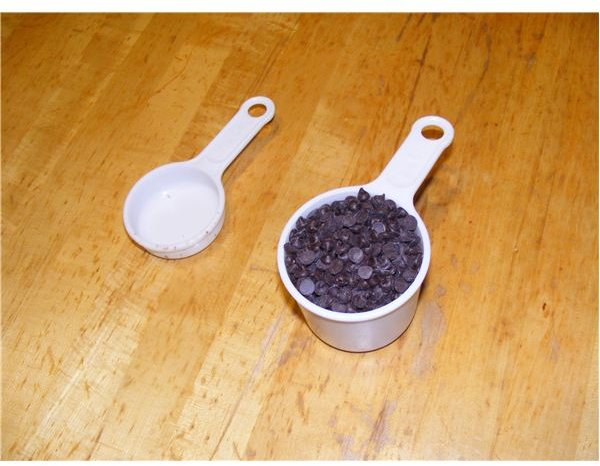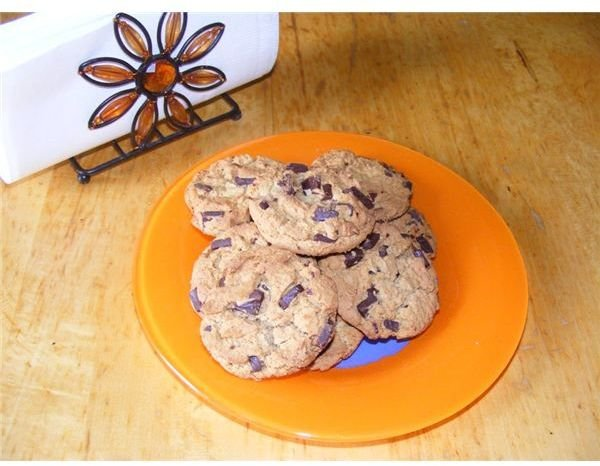# Math Made Easy: Learn the Rules for Multiplying Fractions with Cookies!

Page content

This article will help children to learn to multiply fractions and display what they have learned using a simple hands-on activity. You can use similar activities to multiply proper and improper fractions. By using the results to bake a favorite recipe, the child will be motivated to find the correct answers in an easy way.## Fraction Fun: Doubling a Recipe

To demonstrate multiplying fractions you can motivate your child to double or triple the ingredients of a given recipe. For example in the following chocolate chip recipe, the ingredients are listed as follows:

• 1 cup shortening
• 2/3 cup white sugar
• 2/3 cup brown sugar
• 2 eggs
• 1 ½ cups flour
• 1 tsp salt
• 1 tsp baking soda
• 1 ½ cups chocolate chips
• 1 tsp vanilla
• 1/3 cup chopped nuts
• 2 cups oatmeal

Doubling a Recipe

The student should have no problem seeing they can double the whole numbers - needing 2 cups of shortening, 4 eggs, 2 tsp of salt and baking soda, and 4 cups of oatmeal.

When they need to double the white and brown sugar, 2/3 cup, they may not know how to do it. 2/3 when doubled equals 4/3.To some children, is confusing since it is an improper fraction.

Multiplying That Results in Improper Fractions

It may be easier in recipe making, to take a 1/3 measuring cup and fill it 4 times without explanation. They would write the math sentence down as: 2/3 x 2 = 4/3.

To fully show them how they can double 2/3, they should take the 1/3 measuring cup, twice and fill a larger container, the 1 cup. They will be converting 4/3, the improper fraction to 1 and 1/3.

They can take the 1/3 measuring cup and fill a 1 cup container 3 times, then finding they had another 1/3 cup, they can visualize and demonstrate that 4/3 means filling a 1 cup measuring cup once with 1/3 cup measuring cup also.

They may then understand that a fraction can be doubled by multiplying the numerator only.

Multiplying Mixed Numbers

To see that 1 and ½ cups when doubled means 3 cups, they can fill both a 1 cup and a ½ cup measuring cup and put it into a larger measuring cup. When they do it twice, they will see that it makes 3 cups.

They can see also that you can easily double a whole number. So they can double the 1 cup to make 2 cups. Then they can double the ½ cup by filling it up twice and seeing that it makes 1 cup. By writing the math sentence ½ x 2 they can see that it makes 2/2. The numerator, is doubled. This is the only way it can be 1 cup.

Doubling or tripling recipes means taking the fractions and multiplying the numerators. The students will see that it is easy to multiply the whole numbers and then multiply the numerators of the fractions. Doubling 1 ½ cups made 3 cups.

If they would like to show a fraction written in its improper form such as writing 1 ½ cups as 3/2, this may help them to multiply the numerator only. 3/2 doubled will be 6/2. This is also 3 cups and means dividing the 6 by 2. Students will realize that mixed fractions are actually easier to double when you first multiply the whole numbers, then the fractions.

## Tripling a Recipe

ChallengesTripling or quadrupling a recipe is more of a challenge to a child who wants to understand fractions. Have fun measuring and make a large batch of cookies. In this recipe, 2/3 tripled means 2/3 x 3 or 6/3 or 2 cups.

To understand that multiplying fractions means that the numerator or top number is multiplied, the child should use the 1/3 measuring cup 6 times to see that they are taking 2 thirds, 3 times to fill up 2 full 1 cup measuring cups. Then have the child write the math sentence: 2/3 x 3 = 2

To understand quadrupling, have the child fill a 1/3 measuring cup twice to make 2/3. Then have them do this 4 times. How much in total did it fill? Have them write the math sentence: 2/3 x 4 = 8/3. 8/3 will fill 2 full 1 cup measuring cups and 2/3 of another cup. So, they’ll find that 8/3 = 2 1/3. Then numerator, top number is multiplied. The denominator doesn’t change.

Writing Math Sentences

Have the students write out all the fractions multiplied on paper after they’ve measured the ingredients. This should reinforce multiplying only the numerator, since the container to measure with doesn’t change. The container is the denominator. The denominator doesn’t change.

Check that they have multiplied correctly. Ask them what the result would be in a recipe if they are not multiplying correctly. The importance of measuring the right amount of ingredients will help reinforce why having a correct result is needed in math.

Multiplying fractions usually makes sense to children when they have to apply the concept to a real life situation. To double, triple or quadruple a recipe means the fractional ingredients must be multiplied. The fractions are not always simple fractions such as 1/3. Sometimes the fractions are 2/3 or must be written as improper fractions such as 4/3 in order for the child to easily multiply them.

The important concept in learning to multiply fractions is remembering to multiply only the numerator. This can be reinforced by having the child use only 1 type of measuring cup. Counting or multiplying how many 1/3 cup measuring cups are needed will also help the child to remember that the denominator, or measuring container, doesn’t change when you make a multiple batch from a recipe.

## This post is part of the series: Fraction Fun At Home: Modeling and Writing Fractions With Your Child

This series will show how to write and model fractions with an egg carton at home. Your child will learn to write proper and improper fractions, find equivalent fractions and multiply fractions. It is a fun and easy way to use recipes with fractions for hands on learning.